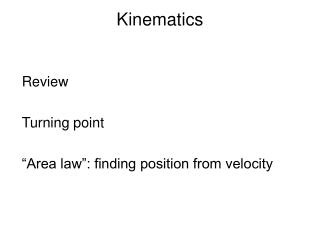DownloadDownload PresentationKinematics

# Kinematics

Télécharger la présentation## Kinematics

- - - - - - - - - - - - - - - - - - - - - - - - - - - E N D - - - - - - - - - - - - - - - - - - - - - - - - - - -
##### Presentation Transcript

1. Kinematics Review Turning point “Area law”: finding position from velocity

2. Q1. Velocity and acceleration • If one is positive the other one has to be positive. • If one is equal zero at some time t, the other has to be zero. • Their signs are independent. • Acceleration is always smaller then velocity.

3. Turning point for 1D motion A point where an object reverses its direction. At a turning point, its velocity is zero. Example: an object is tossed into the air. The initial velocity is +9.8m/s and continues to be positive until it reaches a maximal height at t=1s. Afterwards, its velocity becomes. The turning point in this case is the one with a maximal height.

4. Q2: The turning point in • this graph is • P1; • P2; • 3) P3; • 4) P4.

5. Q3 Turning point t=9s

6. Finding position from velocity Displacement is equal to the area below a velocity curve. The area is positive if the curve is above v=0 axis; negative if below the axis.

7. Motion of a car. A person is driving northward along the east mall Blvd with an initial velocity 10m/s starting from in front of the Hebb theatre. 1) Where is the turning point? 2) Where is the person at t=6s?

8. Q3 The average velocity and average speed between t=0 and 6s in this case are, respectively, • +10m/s and 15m/s; • 8.33 m/s and 8.33 m/s; • - 5m/s and 8.33m/s; • -5m/s and - 8.33m/s.

9. Velocity as a function of time. Calculate the average velocity from such a graph. Average Velocity = area / t